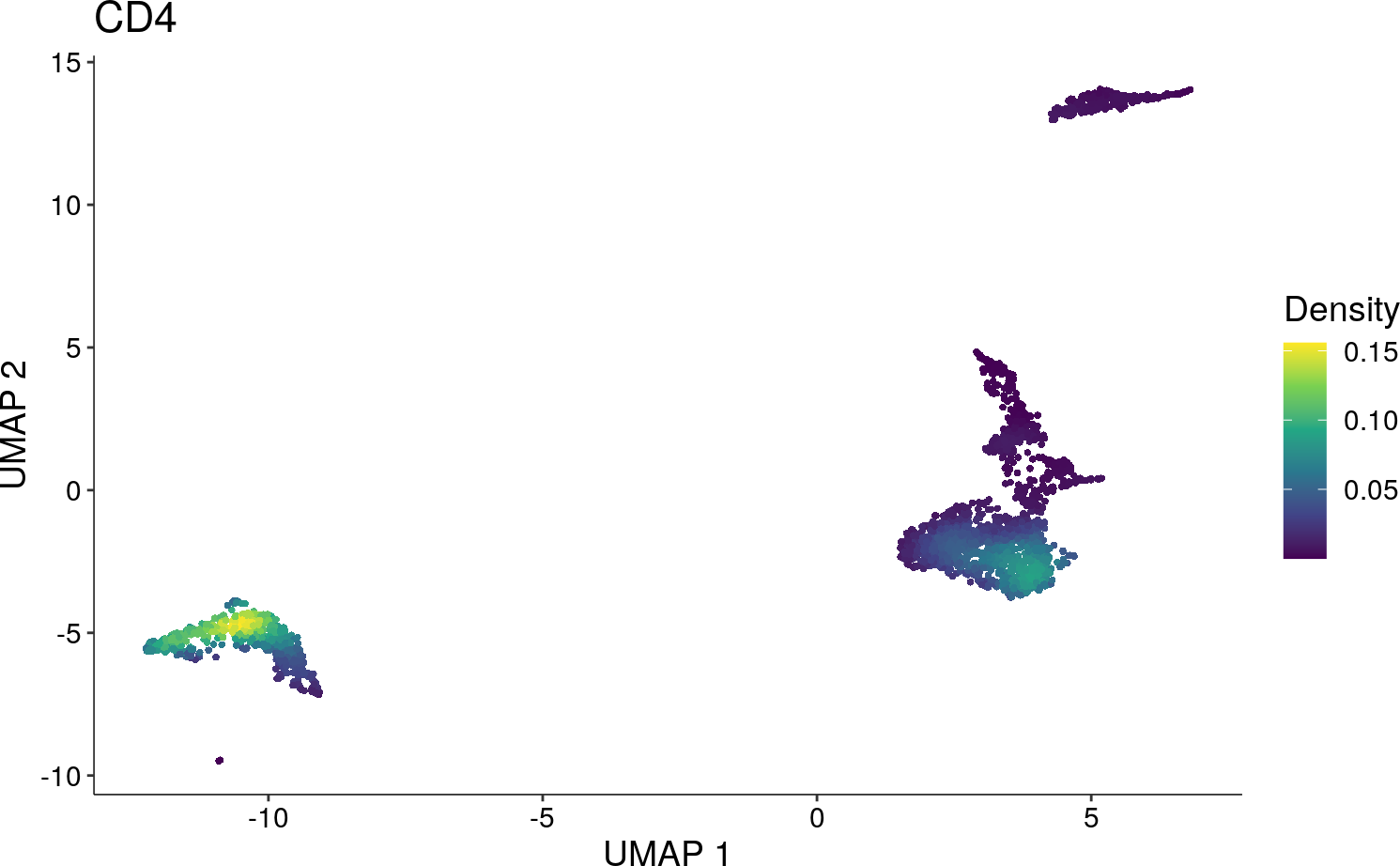# 1 Overview

Due to the sparsity observed in single-cell data (e.g. RNA-seq, ATAC-seq), the visualization of cell features (e.g. gene, peak) is frequently affected and unclear, especially when it is overlaid with clustering to annotate cell types. `Nebulosa` is an R package to visualize data from single cells based on kernel density estimation. It aims to recover the signal from dropped-out features by incorporating the similarity between cells allowing a “convolution” of the cell features.

# 2 Import libraries

For this vignette, let’s use `Nebulosa` with the `scran` and `scater` packages. First, we’ll do a brief/standard data processing.

``````library("Nebulosa")
library("scater")
library("scran")
library("DropletUtils")
library("BiocFileCache")``````

# 3 Data pre-processing

Let’s download a dataset of 3k PBMCs (available from 10X Genomics). For the purpose of this vignette, let’s use the `BiocFileChache` package to dowload the data and store it in a temporary directory defined by the `tempdir()` function. To import the count data, we’ll use the `read10xCounts` from the `DropletUtils` package.

``````bfc <- BiocFileCache(ask = FALSE)
data_file <- bfcrpath(bfc, file.path(
"https://s3-us-west-2.amazonaws.com/10x.files/samples/cell",
"pbmc3k",
"pbmc3k_filtered_gene_bc_matrices.tar.gz"
))

untar(data_file, exdir = tempdir())
"filtered_gene_bc_matrices",
"hg19"
))``````

The default feature names are Ensembl ids, let’s use thegene names and set them as row names of the `sce` object. The following step will use the gene names as rownames and make them unique by appending it’s corresponding Ensemble id when a gene-name duplicate is found.

``````rownames(pbmc) <- uniquifyFeatureNames(rowData(pbmc)[["ID"]],
rowData(pbmc)[["Symbol"]])``````

# 4 Quality control

First, let’s remove features that are not expressed in at least 3 cells.

``````i <- rowSums(counts(pbmc) > 0)
is_expressed <- i > 3
pbmc <- pbmc[is_expressed, ]``````

And cells not expressing at least one UMI in at least 200 genes.

``````i <- colSums(counts(pbmc) > 0)
is_expressed <- i > 200
pbmc <- pbmc[,is_expressed]``````

Finally, let’s remove outlier cells based on the number of genes being expressed in each cell, library size, and expression of mitochondrial genes using the `perCellQCMetrics` and `quickPerCellQC` functions from the `scater` package.

``````is_mito <- grepl("^MT-", rownames(pbmc))
qcstats <- perCellQCMetrics(pbmc, subsets = list(Mito = is_mito))
qcfilter <- quickPerCellQC(qcstats, percent_subsets = c("subsets_Mito_percent"))``````

For more information on quality control, please visit the OSCA website: https://osca.bioconductor.org/quality-control.html

## 4.1 Data normalization

Let’s normalize the data by scaling the counts from each cell across all genes by the sequencing depth of each cell and using a scaling factor of 1 x 10^4. Then, we can stabilize the variance by calculating the pseudo-natural logarithm using the `log1p` function.

``logcounts(pbmc) <- log1p(counts(pbmc) / colSums(counts(pbmc)) * 1e4)``

Please refer to the OSCA website for more details on other normalization strategies: https://osca.bioconductor.org/normalization.html

## 4.2 Dimensionality reduction

A reduced set of variable genes are expected to drive the major differences between the cell populations. To identify these genes, let’s use the `modelGeneVar()` and `getTopHVGsfrom()` from `scran` by selecting the top 3000 most highly-variable genes.

``````dec <- modelGeneVar(pbmc)
top_hvgs <- getTopHVGs(dec, n = 3000)``````

Once the data is normalized and highly-variable features have been determined, we can run a Principal Component Analysis (PCA) to reduce the dimensions of our data to 50 principal components. Then, we can run a Uniform Manifold Approximation and Projection (UMAP) using the principal components to obtain a two-dimensional representation that could be visualized in a scatter plot.

``````set.seed(66)
pbmc <- runPCA(pbmc, scale = TRUE, subset_row = top_hvgs)``````

Finally, we can run the UMAP as follows:

``pbmc <- runUMAP(pbmc, dimred = "PCA")``

## 4.3 Clustering

To assess cell similarity, let’s cluster the data by constructing a Shared Nearest Neighbor (SNN) Graph using the first 50 principal components and applying `cluster_louvain()` from the `igraph` package.

``````g <- buildSNNGraph(pbmc, k = 10, use.dimred = "PCA")
clust <- igraph::cluster_louvain(g)\$membership
colLabels(pbmc) <- factor(clust)``````

# 5 Visualize data with `Nebulosa`

The main function from `Nebulosa` is the `plot_density`.

Let’s plot the kernel density estimate for `CD4` as follows

``plot_density(pbmc, "CD4")``For comparison, let’s also create a standard scatter plot using `scater`

``plotUMAP(pbmc, colour_by = "CD4")``